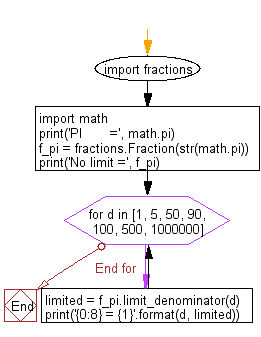﻿ Python Math: Convert a floating point number to an approximate rational value - w3resource# Python Math: Convert a floating point number to an approximate rational value

## Python Math: Exercise-47 with Solution

Write a Python program to convert a floating point number (PI) to an approximate rational value on the various denominator.

Note: max_denominator = 1000000

Sample Solution:-

Python Code:

``````import fractions
import math

print('PI       =', math.pi)

f_pi = fractions.Fraction(str(math.pi))
print('No limit =', f_pi)

for d in [1, 5,  50, 90, 100, 500, 1000000]:
limited = f_pi.limit_denominator(d)
print('{0:8} = {1}'.format(d, limited))
```
```

Sample Output:

```PI       = 3.141592653589793
No limit = 3141592653589793/1000000000000000
1 = 3
5 = 16/5
50 = 22/7
90 = 267/85
100 = 311/99
500 = 355/113
1000000 = 3126535/995207
```

Flowchart:Python Code Editor:

Have another way to solve this solution? Contribute your code (and comments) through Disqus.

What is the difficulty level of this exercise?

Test your Programming skills with w3resource's quiz.

﻿

```>>> students = [{'name': 'John', 'score': 98}, {'name': 'Mike', 'score': 94}, {'name': 'Jennifer', 'score': 99}]